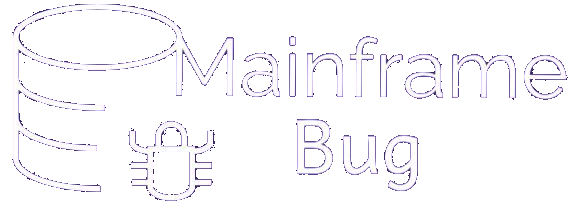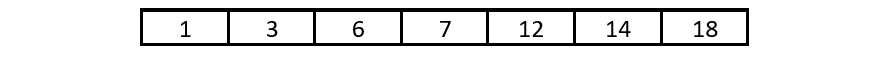## Module 9: Array processing and Table handling

### SEARCH ALL (Binary search)

• Binary search works like this:-
• First of all array on which search is to be made should be in ascending order
• Element to be searched is compared with middle element of array
• If matches, search process ends.
• If search element is less than middle element, array is divided into two halves considering middle element, and search will be done only in first half
• If search element is greater than middle element, only second half will be searched further
• The search operation continues same way in selected half until either element is found or there are no more element to search.
• SEARCH ALL does search operation using binary search method mentioned above
• SEARCH ALL expects that the array on which search is to be made should be in sorted order. Array can be made auto sorted by specifying ASCENDING/DESCENDING KEY option in OCCURS clause as shown below:-

OCCURS integer TIMES [{ASCENDING/DESCENDING} KEY IS data-name-1 [, data-name-2]…] [INDEXED BY index-name-1 [, index-name-2]…]

• When ASCENDING/DESCENDING option is used, array is auto arranged in ascending/descending order whichever is mentioned
• When more than one data name is used, the first one is the first major key and the next is second major key and so on
• #### Binary Search Algorithm:

For example, we are trying to find element “3” in below array:-Important note:- Array should be in ascending order. You can use ASCENDING KEY option of OCCURS clause to achieve it
Step 1: Identify middle element
• Identify total number of element ‘N’ in array or remaining half
• If N is even number, middle element will be at position (N/2)
• If N is odd number, middle element will be at position ((N+1)/2)
• In our case, total number of element is ‘7’, thus middle element will be: ((N+1)/2) = ((7+1)/2) =4
Step 2: Compare search element to middle element and perform below:-
• If search element < middle element
• Divide the array in two halves and perform search in first(left) half by iterating step-1 and step-2
• If search element > middle element
• Divide the array in two halves and perform search in second(right) half by iterating step-1 and step-2
• If search element = middle element
• Success, element found! Terminate search
• In our case 3 < 7, so divide the array into 2 equal halves
• First half will have elements: 1,3,6 and Second half will have elements: 12,14,18
• While doing search in first half in next iteration, binary search will compare first half’s middle element to search element i.e. 3 with 3 and since they are equals, algorithm concludes search element is found and terminates the search
• This kind of search is performed when array is large (100+) as binary search will need less search operation as compared to sequential search.
• Just assume your search element is at 75th position of array (with capacity of 100 elements). In such case sequential search will need 75 search operation, but binary search will need only 3-4 search operations.
• Basic syntax:-

SEARCH ALL array-name     [AT END <imperative-statement-1>]     WHEN data-name-1 IS EQUAL TO          {identifier-1/literal-1/arithmetic-expression-1}          [AND data-name-2 IS EQUAL TO          {identifier-2/literal-2/arithmetic-expression-2}]…          <imperative-statement-2> [END-SEARCH]

• Some important consideration while using SEARCH ALL:-
• array-name must be in sorted order
• Array should have index, but index need not be set to 1
• Only one WHEN clause can be coded
• AT END executes imperative-statement-1 if search element is not found in entire search
• Compound condition(using AND) is allowed
• Example:
• Assume, we have below declaration in DATA DIVISION,

77 ID PIC 9(4). 01 STUDENT-DATA. 05 STUDENT-ARRAY OCCURS 500 TIMES ASCENDING KEY IS STUDENT-ID INDEXED BY S1. 10 STUDENT-ID PIC 9(04). 10 STUDENT-NAME PIC A(15). 10 STUDENT-MARKS PIC 9(03).

• Using binary search, we want to search given ID in STUDENT-ARRAY and display their name and marks. We can use below code for same purpose
• This can be achieved by coding below snippet in PROCEDURE DIVISION,

SEARCH STUDENT-ARRAY AT END DISPLAY ‘STUDENT NOT FOUND’. WHEN ID = STUDENT-ID(S1) DISPLAY ‘STUDENT-NAME:’ STUDENT-NAME(S1) DISPLAY ‘STUDENT-MARKS:’ STUDENT-MARKS(S1)